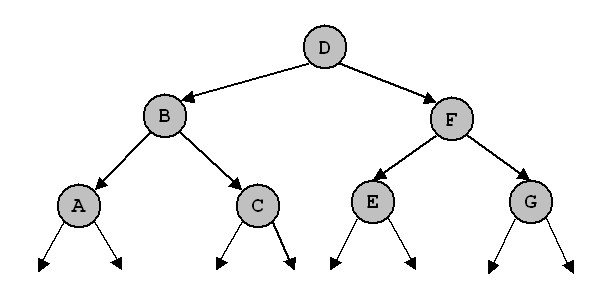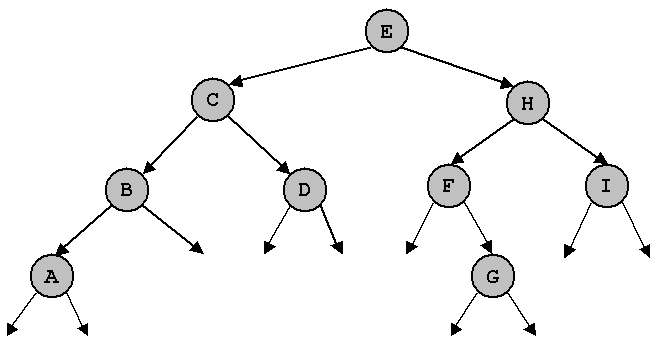```		      EXERCISES ON BINARY TREES

1. Give the inorder and postorder traversal for the tree whose preorder
traversal is A B C - - D - - E - F - -.  The letters correspond to
labeled internal nodes; the minus signs to external nodes.

2. (Sedgewick, Exercise 5.79).  Give the preorder, inorder, postorder,
and level-order traversals of the following binary trees.3. (a)  Write a function that counts the number of items in a binary tree.

(b)  Write a function that returns the sum of all the keys in a binary tree.

(c)  Write a function that returns the maximum value of all the keys in a binary
tree. Assume all values are nonnegative; return -1 if the tree is empty.

4. Write a C function that prints all the keys less than a given value v
in a binary tree.

5. (a)  The height of a tree is the maximum number of nodes on a path from the root
to a leaf node. Write a C function that returns the height of a binary tree.

(b)  The cost of a path in a tree is sum of the keys of the nodes participating
in that path. Write a C function that returns the cost of the most expensive
path from the root to a leaf node.

6. A binary tree is said to be "balanced" if both of its subtrees
are balanced and the height of its left subtree differs from the
height of its right subtree by at most 1.  Write a C function to
determine whether a given binary tree is balanced.

7. What value does the following C function return when called with each of the
binary trees in question 2?